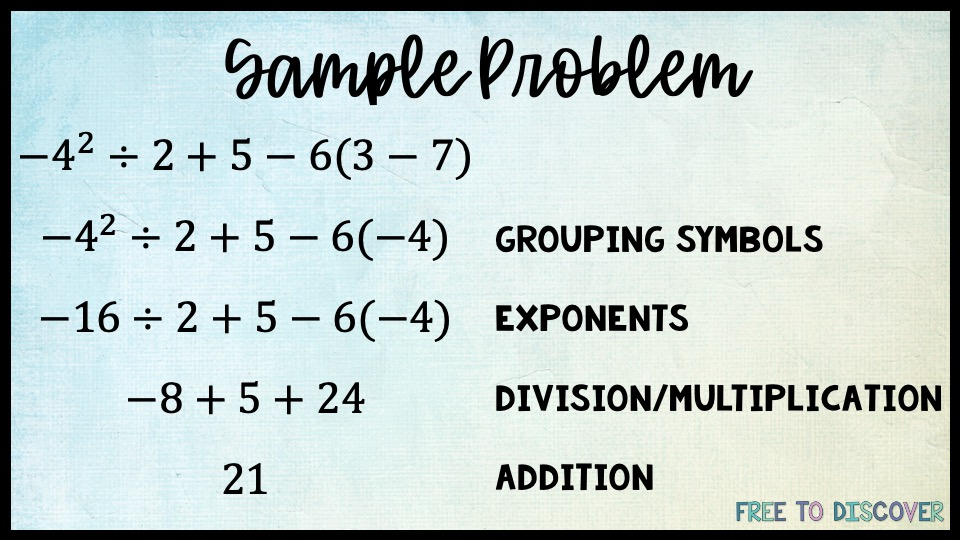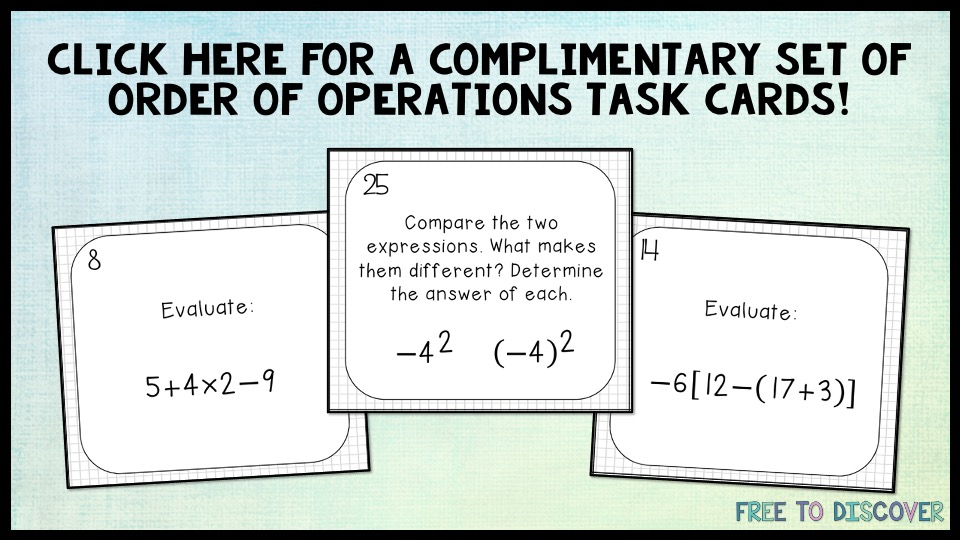# An Order of Operations Brainbuster

Foundational to mathematics, order of operations is an integral topic is math education. There is widespread agreement in teaching either PEMDAS (parenthesis, exponents, multiplication/division, addition/subtraction) or GEMS (groupings, exponents, multiplication/division, subtraction/addition). However, there is one case in particular that leaves students and teachers alike scratching their heads: -nis the not the same as (-n)2.

PSA: Parentheses matter.

###### Let's break this down generally first.

The expression -n2 has no grouping symbols, which means exponents would come next. In this case, the negative in front serves as a factor of -1. In other words, we can write the expression like this: -1n2. Including the 1 can help students see that the base will be squared before multiplying by -1.

The expression (-n)does have grouping symbols, which means everything in parenthesis will be squared. In this case, the expression can be rewritten as (-n)(-n) or just n2.

The difference is in the sign of the coefficient.

###### Now let's look at a specific case.

-32 means (-1)3so (-1)9 = -9.

(-3)means (-3)(-3) = 9.

###### Here is the concept within an order of operations practice problem.So remember, parentheses matter! Because of this, it is very important that when students evaluate an expression using substitution they use parentheses. Given xand x=-2, (-2)2=4. Because x is being squared here, the sign is included in the operation. However, given -xand x=2, -(2)2=-4.What other tricky cases should we explore in the future?

#### Join the Free to Discover community!

When you subscribe, you'll access our FREE monthly newsletter for secondary math students!